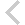FICO Xpress Optimization Examples Repository
 FICO Optimization Community FICO Xpress Optimization HomeIntroductory examples

Description
approx burglar chess Problem name and type, features Difficulty Approximation: Piecewise linear approximation ** SOS-2, Special Ordered Sets, piecewise linear approximation of a nonlinear function MIP modeling: Knapsack problem: 'Burglar' * simple MIP model with binary variables, data input from text data file, array initialization, numerical indices, string indices, record data structure LP modeling: Production planning: 'Chess' problem * simple LP model, solution output, primal solution values, slack values, activity values, dual solution values All item discount pricing: Piecewise linear function *** SOS-1, Special Ordered Sets, piecewise linear function, approximation of non-continuous function, step function Incremental pricebreaks: Piecewise linear function *** SOS-2, Special Ordered Sets, piecewise linear function, step function

Further explanation of this example: 'Applications of optimization with Xpress-MP', Introductory examples (Chapters 1 to 5) of the book 'Applications of optimization with Xpress-MP'

Source Files

Data Files

burglari.mos

```(!******************************************************
Mosel Example Problems
======================

file burglari.mos
`````````````````
Knapsack problem
-- Using string indices --

(c) 2008 Fair Isaac Corporation
author: R.C. Daniel, Jul. 2002
*******************************************************!)

model "Burglar 1 (index set)"
uses "mmxprs"

declarations
ITEMS = {"camera", "necklace", "vase", "picture", "tv", "video",
"chest", "brick"}     ! Set for items
WTMAX = 102                    ! Maximum weight allowed

VALUE: array(ITEMS) of real    ! Value of items
WEIGHT: array(ITEMS) of real   ! Weight of items

take: array(ITEMS) of mpvar    ! 1 if we take item i; 0 otherwise
end-declarations

VALUE("camera")  := 15;  WEIGHT("camera")  :=  2
VALUE("necklace"):=100;  WEIGHT("necklace"):= 20
VALUE("vase")    := 90;  WEIGHT("vase")    := 20
VALUE("picture") := 60;  WEIGHT("picture") := 30
VALUE("tv")      := 40;  WEIGHT("tv")      := 40
VALUE("video")   := 15;  WEIGHT("video")   := 30
VALUE("chest")   := 10;  WEIGHT("chest")   := 60
VALUE("brick")   :=  1;  WEIGHT("brick")   := 10

(! Alternative data initialization:
VALUE :: (["camera", "necklace", "vase", "picture", "tv", "video",
"chest", "brick"])[15, 100, 90, 60, 40, 15, 10, 1]
WEIGHT:: (["camera", "necklace", "vase", "picture", "tv", "video",
"chest", "brick"])[ 2,  20, 20, 30, 40, 30, 60, 10]
!)

! Objective: maximize total value
MaxVal:= sum(i in ITEMS) VALUE(i)*take(i)

! Weight restriction
sum(i in ITEMS) WEIGHT(i)*take(i) <= WTMAX

! All variables are 0/1
forall(i in ITEMS) take(i) is_binary

maximize(MaxVal)                 ! Solve the MIP-problem

! Print out the solution
writeln("Solution:\n Objective: ", getobjval)
forall(i in ITEMS)  writeln(" take(", i, "): ", getsol(take(i)))
end-model

```